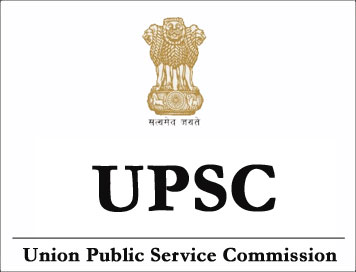NEW!

# (Syllabus) Indian Statistical Service Examination (ISS) - STATISTICS - I## STATISTICS-I

1. Probaility:  Elements of measure theory, Classical definitions and axiomatic approach. Sample space. Class of events and Probability measure. Laws of total and compound probability. Probability of m events out of n. Conditional probability, Bayes’ theorem. Random variables - discrete and continuous. Distribution function. Standard probability distributions - Bernoulli, uniform, binomial, Poisson, geometric, rectangular, exponential, normal, Cauchy, hypergeometric, multinomial, Laplace, negative binomial, beta, gamma, lognormal and compound. Poisson distribution. Joint distributions, conditional distributions, Distributions of functions of random variables. Convergence in distribution, in probability, with probability one and in mean square. Moments and cumulants. Mathematical expectation and conditional expectation. Characteristic function and moment and probability generating functions Inversion uniqueness and continuity theorems. Borel 0-1 law: Kolmogorov’s 0-1 law. Tchebycheff’s and Kolmogorov’s inequalities. Laws of large numbers and central limit theorems for independent variables. Conditional expectation and Martingales.

2. Statistical Methods: (a) Collection, compilation and presentation of data, Charts, diagrams and histogram. Frequency distribution. Measures of location, dispersion, skewness and kurtosis. Bivariate and multivariate data. Association and contingency. Curve fitting and orthogonal polynomials. Bivariate normal distribution. regression-linear, polynomial. Distribution of the correlation coefficient, Partial and multiple correlation, Intraclass correlation, Correlation ratio.(b) Standard errors and large sample test. Sampling distributions of x,s2, t, chisqure and F; tests of significance based on them, Small sample tests. (c) Non-parametric tests-Goodness of fit, sign, median, run, Wicloxon, Mann-Whitney, Wald-Wolfowitz and Kolmogorov-Smirnov. Rank order statistics-minimum, maximum, range and median. Concept of Asymptotic relative effciency. 3. Numerical Analysis Interpolation formulae (with remainder terms) due to Lagrange, Newton-Gregory, Newton Divided different, Gauss and Striling. Euler-Maclaurin’s summation formula. Inverse interpolation. Numerical integration and differentiation. Difference equations of the first order. Linear difference equations with constant coefficients.